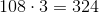## Example Questions

### Example Question #1 : How To Find An Angle In A Pentagon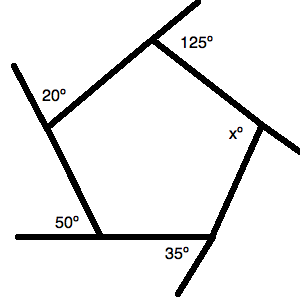The figure above is a pentagon.  All of the angles listed (except the interior one) are exterior angles to the pentagon's interior angles.

What is the value ofin the figure above?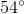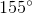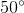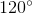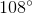Explanation:

There are two key things for a question like this. The first is to know that a polygon has a total degree measure of: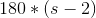, whereis the number of sides.

Therefore, a hexagon like this one has: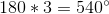Next, you should remember that all of the exterior angles listed are supplementary to their correlative interior angles. This lets you draw the following figure: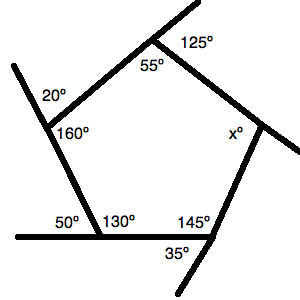Now, you just have to manage your algebra well. You must sum up all of the interior angles and set them equal to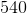. Thus, you can write: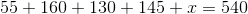Solve for: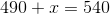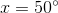### Example Question #2 : How To Find An Angle In A Pentagon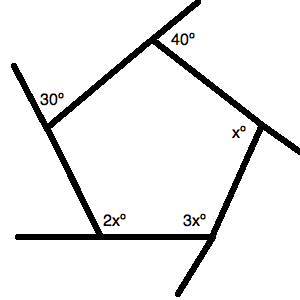The figure above is a pentagon.  All of the angles listed (except the interior one) are exterior angles to the pentagon's interior angles.

What is the value of the largest unknown angle in the figure above?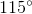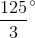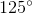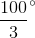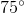Explanation:

There are two key things for a question like this. The first is to know that a polygon has a total degree measure of:, whereis the number of sides.

Therefore, a hexagon like this one has:.

Next, you should remember that all of the exterior angles listed are supplementary to their correlative interior angles. This lets you draw the following figure: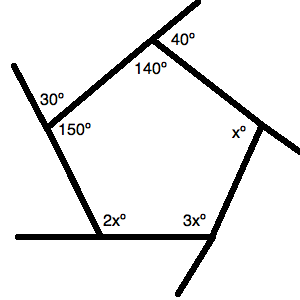Now, you just have to manage your algebra well. You must sum up all of the interior angles and set them equal to. Thus, you can write: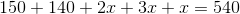Solve for: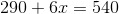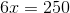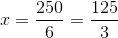Now, you have to find the largest unknown angle, which is: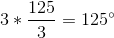### Example Question #3 : How To Find An Angle In A Pentagon

What is the sum of three angles in a pentagon?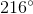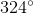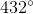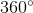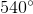Explanation:

The sum of all angles is determined by the following formula for a polygon: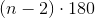In a pentagon, there are 5 sides, or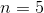.  Substitute and find the total possible angle in a pentagon.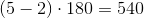There are 5 interior angles in a pentagon.  Divide the total possible angle by 5 to determine the value of one interior angle.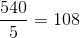Each interior angle of a pentagon is 108 degrees.

The sum of three angles in a pentagon is: